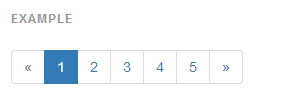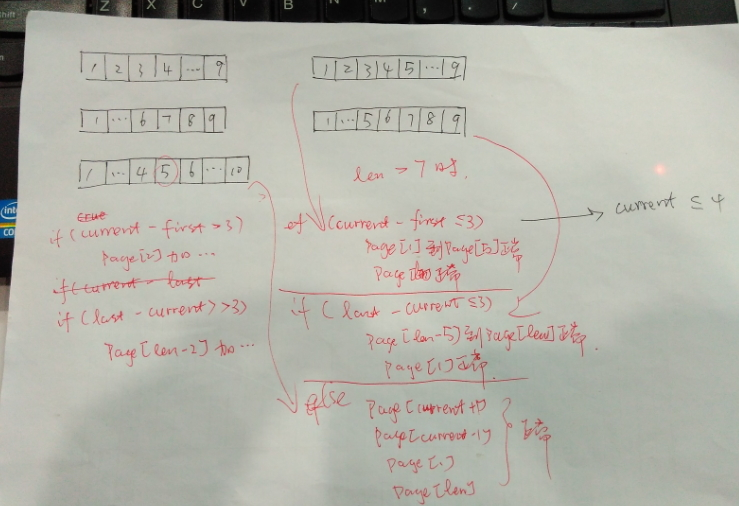Spring Data + Thymeleaf 3 + Bootstrap 4 实现分页器

2
12
01. 云栖社区>
2. 博客>
3. 正文

Spring Data + Thymeleaf 3 + Bootstrap 4 实现分页器

waylau 2017-03-13 00:52:36 浏览6683

分页器有哪些需求

• 显示页码的列表；
• 该列表的第一项是“上一页”，最后一项是“下一页”；
• 当前选中的页码要高亮；
• 当当前页的上一页没有页码可选时，则“上一页”置为不可点击的状态；
• 当当前页的下一页没有页码可选时，则“下一页”置为不可点击的状态；Spring Data 能做什么

org.springframework.data.domain.Page 是 Spring Data 提供的一个分页器接口，提供了常用的方法，比如：

• List getContent(); // 返回分页后的数据的列表
• int getTotalPages(); // 总页数
• long getTotalElements(); // 总数据量
• boolean isFirst(); // 是否是第一个数据；
• boolean isLast(); // 是否是最后一个数据；
• int getNumber(); // 当前页面索引

Spring Data 可以说提供了我们前端分页器所需要的所有元素了。

Thymeleaf 牛刀小试

Thymeleaf 作为模版引擎，其好处就是可以绑定数据源，并且根据数据源来渲染页面。最爽的莫过于根据绑定的数据列表来遍历生成页面元素，比如：

<ul class="pagination" >
<!-- 上一页 -->
<li class="page-item" data-th-classappend="*{first} ? 'disabled' : ''">
<a href="javascript:void(0);" class="page-link" data-th-attr="pageIndex=${page.number} - 1" aria-label="Previous"> <span aria-hidden="true">«</span> </a> </li> <!-- 迭代生成页码 --> <li class="page-item" data-th-each="i :${#numbers.sequence(1, page.totalPages)}"
data-th-classappend="${(page.number + 1) eq i} ? 'active' : ''" > <a class="page-link" data-th-attr="pageIndex=${i} - 1" href="javascript:void(0);">
<span data-th-text="${i}"></span> </a> </li> <!-- 下一页 --> <li class="page-item" data-th-classappend="*{last} ? 'disabled' : ''"> <a href="javascript:void(0);" class="page-link" data-th-attr="pageIndex=${page.number} + 1" aria-label="Next">
<span aria-hidden="true">»</span>
</a>
</li>
</ul>

考虑的再多一点

• 当当前页页码接近首页时，省略号在后部出现；
• 当当前页页码接最后页时，省略号在前部出现；
• 最烦的要属于，当当前页在中部时，前部、后部都需要省略号；

带省略号的分页器• “上一页”和“下一页”的算法于我们上面的简单版本类似，这里就不赘述了。
• 当前页面页码小于等于4时，省略号在列表后部的倒数第二个出现；
• 最后一页与当前页面之差小于等于3时，省略号在列表前部的第二个位置出现；
• 其余情况，则当前页适中处于中间位置，省略号同时在列表第二个位置及倒数第二个位置出现。

<!-- 处理页数大于7 的情况 -->
<ul class="pagination" data-th-if="${page.totalPages gt 7}" > <!-- 上一页 --> <li class="page-item" data-th-classappend="*{first} ? 'disabled' : ''"> <a href="javascript:void(0);" class="page-link" data-th-attr="pageIndex=${page.number} - 1" aria-label="Previous">
<span aria-hidden="true">«</span>
</a>
</li>

<!-- 首页 -->
<li class="page-item" data-th-classappend="${(page.number + 1) eq 1} ? 'active' : ''" > <a href="javascript:void(0);" class="page-link" data-th-attr="pageIndex=0">1</a> </li> <!-- 当前页面小于等于4 --> <li class="page-item" data-th-if="${(page.number + 1) le 4}" data-th-each="i : ${#numbers.sequence(2,5)}" data-th-classappend="${(page.number + 1) eq i} ? 'active' : ''" >
<a class="page-link" href="javascript:void(0);" data-th-attr="pageIndex=${i} - 1"> <span data-th-text="${i}"></span>
</a>
</li>

<li class="page-item disabled" data-th-if="${(page.number + 1) le 4}"> <a href="javascript:void(0);" class="page-link"> <span aria-hidden="true">...</span> </a> </li> <!-- 最后一页与当前页面之差，小于等于3 --> <li class="page-item disabled" data-th-if="${(page.totalPages-(page.number + 1)) le 3}">
<span aria-hidden="true">...</span>
</a>
</li>
<li class="page-item" data-th-if="${(page.totalPages-(page.number + 1)) le 3}" data-th-each="i :${#numbers.sequence(page.totalPages-4, page.totalPages-1)}"
data-th-classappend="${(page.number + 1) eq i} ? 'active' : ''" > <a class="page-link" href="javascript:void(0);" data-th-attr="pageIndex=${i} - 1">
<span data-th-text="${i}"></span> </a> </li> <!-- 最后一页与当前页面之差大于3，且 当前页面大于4--> <li class="page-item disabled" data-th-if="${((page.number + 1) gt 4) && ((page.totalPages-(page.number + 1)) gt 3 )}">
<span aria-hidden="true">...</span>
</a>
</li>
<li class="page-item" data-th-if="${((page.number + 1) gt 4) && ((page.totalPages-(page.number + 1)) gt 3 )}" > <a href="javascript:void(0);" class="page-link" data-th-attr="pageIndex=${page.number}">[[${page.number}]]</a> </li> <li class="page-item active" data-th-if="${((page.number + 1) gt 4) && ((page.totalPages-(page.number + 1)) gt 3 )}">
<a href="javascript:void(0);" class="page-link" data-th-attr="pageIndex=${page.number} + 1">[[${page.number + 1}]]</a>
</li>
<li class="page-item" data-th-if="${((page.number + 1) gt 4) && ((page.totalPages-(page.number + 1)) gt 3 )}"> <a href="javascript:void(0);" class="page-link" data-th-attr="pageIndex=${page.number} + 2">[[${page.number + 2}]]</a> </li> <li class="page-item disabled" data-th-if="${((page.number + 1) gt 4) && ((page.totalPages-(page.number + 1)) gt 3 )}">
<span aria-hidden="true">...</span>
</a>
</li>

<!-- 最后一页 -->
<li class="page-item" data-th-classappend="${(page.number + 1) eq page.totalPages} ? 'active' : ''" > <a href="javascript:void(0);" class="page-link" data-th-attr="pageIndex=${page.totalPages} - 1">[[${page.totalPages}]]</a> </li> <!-- 下一页 --> <li class="page-item" data-th-classappend="*{last} ? 'disabled' : ''"> <a href="javascript:void(0);" class="page-link" data-th-attr="pageIndex=${page.number} + 1" aria-label="Next">
<span aria-hidden="true">»</span>
</a>
</li>
</ul>

还要再考虑的多一点？

• 是否可以选择页面的最大页？
• 是否可以选择任意页面的索引？
• ...

waylau
+ 关注

corcosa 11086人浏览